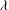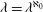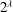Hostname: page-component-5db6c4db9b-wnbrb Total loading time: 0 Render date: 2023-03-26T19:50:15.553Z Has data issue: true Feature Flags: { "useRatesEcommerce": false } hasContentIssue true

# FREE GROUPS AND AUTOMORPHISM GROUPS OF INFINITE STRUCTURES

Published online by Cambridge University Press:  17 April 2014

## Abstract

HTML view is not available for this content. However, as you have access to this content, a full PDF is available via the ‘Save PDF’ action button.

Given a cardinal$\lambda$ with$\lambda =\lambda ^{\aleph _0}$, we show that there is a field of cardinality$\lambda$ whose automorphism group is a free group of rank$2^\lambda$. In the proof of this statement, we develop general techniques that enable us to realize certain groups as the automorphism group of structures of a given cardinality. They allow us to show that analogues of this result hold for free objects in various varieties of groups. For example, the free abelian group of rank$2^\lambda$ is the automorphism group of a field of cardinality$\lambda$ whenever$\lambda$ is a cardinal with$\lambda =\lambda ^{\aleph _0}$. Moreover, we apply these techniques to show that consistently the assumption that$\lambda =\lambda ^{\aleph _0}$ is not necessary for the existence of a field of cardinality$\lambda$ whose automorphism group is a free group of rank$2^\lambda$. Finally, we use them to prove that the existence of a cardinal$\lambda$ of uncountable cofinality with the property that there is no field of cardinality$\lambda$ whose automorphism group is a free group of rank greater than$\lambda$ implies the existence of large cardinals in certain inner models of set theory.

## MSC classification

Type
Research Article
Information
Forum of Mathematics, Sigma , 01 July 2014 , e8
Creative Commons## References

Baumgartner, J. E., ‘Applications of the proper forcing axiom’, inHandbook of set-theoretic topology (North-Holland, Amsterdam, 1984), 913959.CrossRefGoogle Scholar
Baumgartner, J. E., ‘Iterated forcing’, inSurveys in Set Theory, London Math. Soc. Lecture Note Ser., 87 (Cambridge Univ. Press, Cambridge, 1983), 159.Google Scholar
de Bruijn, N. G., ‘Embedding theorems for infinite groups’, Nederl. Akad. Wetensch. Proc. Ser. A. 60 = Indag. Math. 19 (1957), 560569.CrossRefGoogle Scholar
Fried, E. and Kollár, J., ‘Automorphism groups of fields’, inUniversal Algebra (Esztergom, 1977), Colloq. Math. Soc. János Bolyai, 29 (North-Holland, Amsterdam, 1982), 293303.Google Scholar
Hamkins, J. D., ‘Extensions with the approximation and cover properties have no new large cardinals’, Fund. Math. 180 (3) (2003), 257277.CrossRefGoogle Scholar
Hodges, W., Model Theory, Encyclopedia of Mathematics and its Applications, 42 (Cambridge University Press, Cambridge, 1993).CrossRefGoogle Scholar
Jech, T., ‘More game-theoretic properties of Boolean algebras’, Ann. Pure Appl. Logic. 26 (1) (1984), 1129.CrossRefGoogle Scholar
Jech, T., Set Theory, Springer Monographs in Mathematics (Springer-Verlag, Berlin, 2003), The third millennium edition, revised and expanded.Google Scholar
Jensen, R. and Steel, J., ‘${\rm K}$ without the measurable’, J. Symbolic Logic. 78 (3) (2013), 708734.CrossRefGoogle Scholar
Just, W., Shelah, S. and Thomas, S., ‘The automorphism tower problem revisited’, Adv. Math. 148 (2) (1999), 243265.CrossRefGoogle Scholar
Kaplan, I. and Shelah, S., ‘Automorphism towers and automorphism groups of fields without choice’, inGroups and Model Theory, Contemp. Math, 576 (Amer. Math. Soc., Providence, RI, 2012), 187203.CrossRefGoogle Scholar
Mac Lane, S., Categories for the Working Mathematician, 2nd edn, Graduate Texts in Mathematics, 5 (Springer-Verlag, New York, 1998).Google Scholar
Mitchell, W., ‘Aronszajn trees and the independence of the transfer property’, Ann. Math. Logic. 5 (1972/73), 2146.CrossRefGoogle Scholar
Sanerib, R., ‘Automorphism groups of ultrafilters’, Algebra Universalis. 4 (1974), 141150.CrossRefGoogle Scholar
Schimmerling, E., ‘A core model toolbox and guide’, inHandbook of Set Theory Vols. 1, 2, 3 (Springer, Dordrecht, 2010), 16851751.CrossRefGoogle Scholar
Shelah, S., ‘Applications of PCF theory’, J. Symbolic Logic. 65 (4) (2000), 16241674.CrossRefGoogle Scholar
Shelah, S., ‘A countable structure does not have a free uncountable automorphism group’, Bull. London Math. Soc. 35 (1) (2003), 17.CrossRefGoogle Scholar
Shelah, S., Cardinal arithmetic, Oxford Logic Guides, 29 (Oxford University Press, New York, 1994), Oxford Science Publications.Google Scholar
Solecki, S., ‘Polish group topologies’, inSets and Proofs Leeds, 1997, London Math. Soc. Lecture Note Ser., 258 (Cambridge University Press, Cambridge, 1999), 339364.Google Scholar